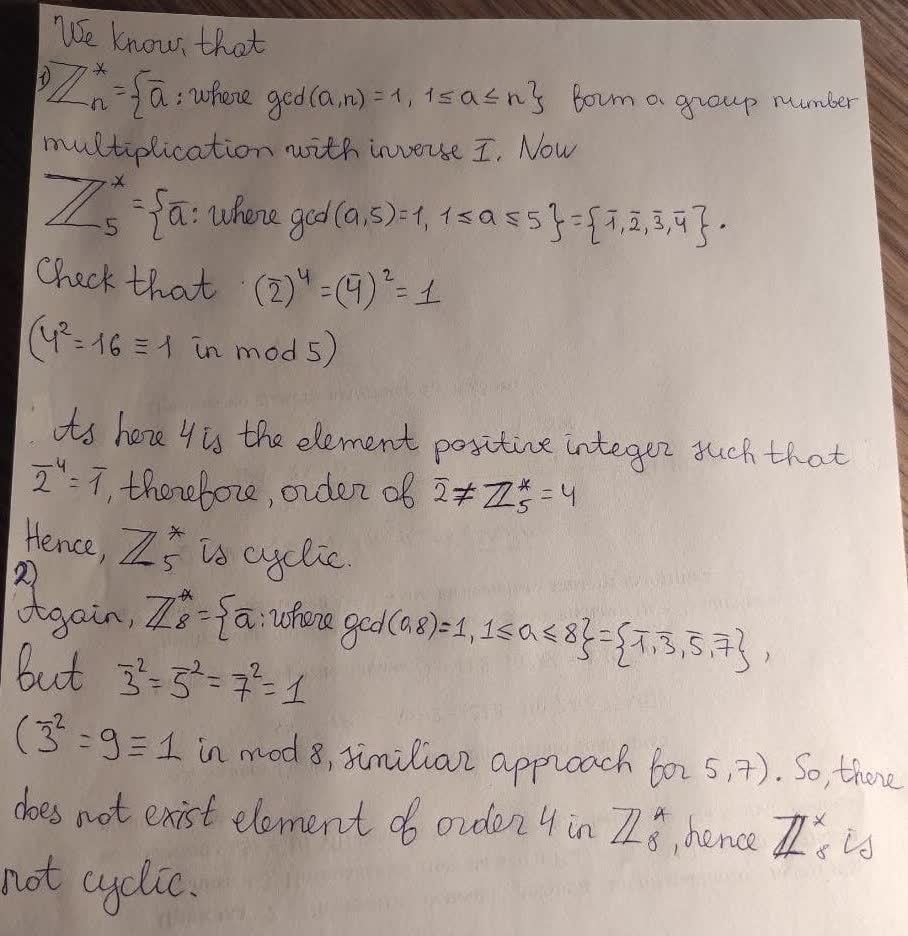# Prove the following.(1) Z ∗ 5 is a cyclic group. (2) Z ∗ 8 is not a cyclic group.he298c 2021-02-27 Answered

Prove the following.
(1) $Z×5$ is a cyclic group.
(2) $Z×8$ is not a cyclic group.

You can still ask an expert for help

• Questions are typically answered in as fast as 30 minutes

Solve your problem for the price of one coffee

• Math expert for every subject
• Pay only if we can solve itIsma Jimenez

1) We know that form a froup under multiplication with inverse bar1. Now,

Check that
${\left(\stackrel{―}{2}\right)}^{4}={\left(\stackrel{―}{4}\right)}^{2}=1,$
$\left({4}^{2}=16\equiv \in \text{mod}5\right)$
As here 4 is the element positive integer such that ${\stackrel{―}{2}}^{4}=\stackrel{―}{1}$, therefore, order of $\stackrel{―}{2}\ne {\mathbb{Z}}_{5}^{\ast }=4$. Hence, ${\mathbb{Z}}_{5}^{\ast }$ is cyclic
2) Again
but ${\overline{3}}^{2}={\overline{5}}^{2}={\overline{7}}^{2}=\overline{1}$
(${3}^{2}=9\equiv 1\in \text{mod}8$, similar approach for 5,7). So, there does not exists any element of order $4\in {\mathbb{Z}}_{8}^{\ast }$, hence ${\mathbb{Z}}_{8}^{\ast }$ is not cyclic.